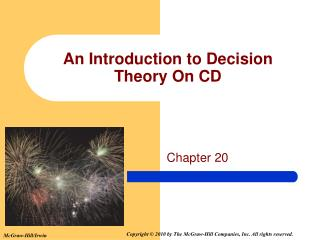Download PresentationAn Introduction to Decision Theory On CD

Loading in 2 Seconds...

# An Introduction to Decision Theory On CD - PowerPoint PPT Presentation

An Introduction to Decision Theory On CD. Chapter 20. GOALS. Define the terms state of nature, event, decision alternative, and payoff . Organize information in a payoff table or a decision tree. Find the expected payoff of a decision alternative.I am the owner, or an agent authorized to act on behalf of the owner, of the copyrighted work described.
Download Presentation## An Introduction to Decision Theory On CD

An Image/Link below is provided (as is) to download presentation

Download Policy: Content on the Website is provided to you AS IS for your information and personal use and may not be sold / licensed / shared on other websites without getting consent from its author.While downloading, if for some reason you are not able to download a presentation, the publisher may have deleted the file from their server.

- - - - - - - - - - - - - - - - - - - - - - - - - - E N D - - - - - - - - - - - - - - - - - - - - - - - - - -
Presentation Transcript
1. An Introduction to Decision Theory On CD Chapter 20

2. GOALS • Define the terms state of nature, event, decision alternative, and payoff. • Organize information in a payoff table or a decision tree. • Find the expected payoff of a decision alternative. • Compute opportunity loss and expected opportunity loss. • Assess the expected value of information.

3. Statistical Decision Theory • Classical statistics focuses on estimating a parameter, such as the population mean, constructing confidence intervals, or hypothesis testing. • Statistical Decision Theory (Bayesian statistics) is concerned with determining which decision, from a set of possible decisions, is optimal. Three components to any decision-making situation: • The available choices (alternatives or acts). • The states of nature, which are not under the control of the decision maker - uncontrollable future events. • The payoffs - needed for each combination of decision alternative and state of nature. • A Payoff Table is a listing of all possible combinations of decision alternatives and states of nature. • The Expected Payoff or the Expected Monetary Value (EMV) is the expected value for each decision.

4. Decision Making

5. Calculating the EMV • Let Ai be the ithdecision alternative. • Let P(Sj) be the probability of the jth state of nature. • Let V(Ai, Sj) be the value of the payoff for the combination of decision alternative Ai and state of nature Sj. • Let EMV (Ai) be the expected monetary value for the decision alternative Ai.

6. (.60) (.40) Decision Making Under Conditions of Uncertainty - Example EXAMPLE Bob Hill, a small investor, has \$1,100 to invest. He has studied several common stocks and narrowed his choices to three, namely, Kayser Chemicals, Rim Homes, and Texas Electronics. He estimated that, if his \$1,100 were invested in Kayser Chemicals and a strong bull market developed by the end of the year (that is, stock prices increased drastically), the value of his Kayser stock would more than double, to \$2,400. However, if there were a bear market (i.e., stock prices declined), the value of his Kayser stock could conceivably drop to \$1,000 by the end of the year. His predictions regarding the value of his \$1,100 investment for the three stocks for a bull market and for a bear market are shown below. A study of historical records revealed that during the past 10 years stock market prices increased six times and declined only four times. According to this information, the probability of a market rise is .60 and the probability of a market decline is .40. Calculation (A1)=(.6)(\$2,400)+(.4)(\$1,000) =\$1,840 (A2)=(.6)(\$2,400)+(.4)(\$1,000) =\$1,760 (A3)=(.6)(\$2,400)+(.4)(\$1,000) =\$1,600

7. Opportunity Loss Opportunity Loss or Regret is the loss because the exact state of nature is not known at the time a decision is made. • The opportunity loss is computed by taking the difference between the optimal decision for each state of nature and the other decision alternatives. Opportunity Loss when Market Declines Kayser: \$1,150 - \$1,000= \$150 Rim Homes: \$1,150 - \$1,100 = \$50 Texas Electronics: \$1,150 - \$1,150 = \$0 Opportunity Loss when Market Rises Kayser: \$2,400 - \$2,400= \$0 Rim Homes: \$2,400 - \$2,200 = \$200 Texas Electronics: \$2,400 - \$1,900 = \$500

8. 0.40 0.60 Expected Opportunity Loss (A1)=(.6)(\$0)+(.4)(\$150) =\$60 (A2)=(.6)(\$200)+(.4)(\$50) =\$140 (A3)=(.6)(\$500)+(.4)(\$0) =\$300

9. Payoff Table Opportunity Loss Table Maximin, Maximax, andMinimax Regret Strategies Maximin strategy maximizes the minimum gain. It is a pessimistic strategy. Maximax strategy maximizes the maximum gain. Opposite of a maximin approach, it is an optimistic strategy Minimax regret strategy minimizes the maximum regret (opportunity loss). This is another pessimistic strategy Maximin 1,000 1,100 1,150 Maximax 2,400 2,200 1,900 Minimax Regret 150 200 500

10. Step 1: Compute the Expected Value Under Certainty (.60) (.40) Expected Value Under Certainty Step 2: Compute the Expected Value Under Uncertainty Value of Perfect Information What is the worth of information known in advance before a strategy is employed? Expected Value of Perfect Information (EVPI) is the difference between the expected payoff if the state of nature were known and the optimal decision under the conditions of uncertainty. Step 3: Subtract the Expected Value Under Uncertainty from the Expected Value Under Certainty

11. Sensitivity Analysis and Decision Trees Sensitivity Analysis examines the effects of various probabilities for the states of nature on the expected values for the decision alternatives. Decision Trees are useful for structuring the various alternatives. A decision tree is a picture of all the possible courses of action and the consequent possible outcomes. • A box is used to indicate the point at which a decision must be made, • The branches going out from the box indicate the alternatives under consideration \$2,400